# First integral

(diff) ← Older revision | Latest revision (diff) | Newer revision → (diff)

of an ordinary differential equation

A non-constant continuously-differentiable function whose derivative vanishes identically on the solutions of that equation. For a scalar equation(*)

a first integral is a function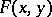which occurs in the general solution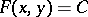, whereis an arbitrary constant. Therefore,satisfies the linear equation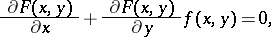containing first-order partial derivatives. A first integral need not exist throughout the domain of definition of (*), but it always exists in a small region around a point at which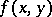is continuously differentiable. A first integral is not uniquely defined. For example, for the equation, a first integral is not onlybut also, e.g.,.

Knowledge of a first integral for a normal system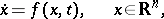enables one to reduce the order of this system by one, while the search forfunctionally-independent first integrals is equivalent to the search for the general solution in implicit form. Ifare functionally-independent first integrals, then any other first integralcan be put in the formwhereis some differentiable function.

How to Cite This Entry:
First integral. Encyclopedia of Mathematics. URL: http://encyclopediaofmath.org/index.php?title=First_integral&oldid=12974
This article was adapted from an original article by N.N. Ladis (originator), which appeared in Encyclopedia of Mathematics - ISBN 1402006098. See original article